Maths
Study Material

# Mean, Median and Mode of grouped data – Discrete

Learn to calculate mean, median and mode of discrete grouped data. Take a look at the solved problems for better understanding of the concept.

5 minutes long
Posted by Tanwir Silar, 18/8/2021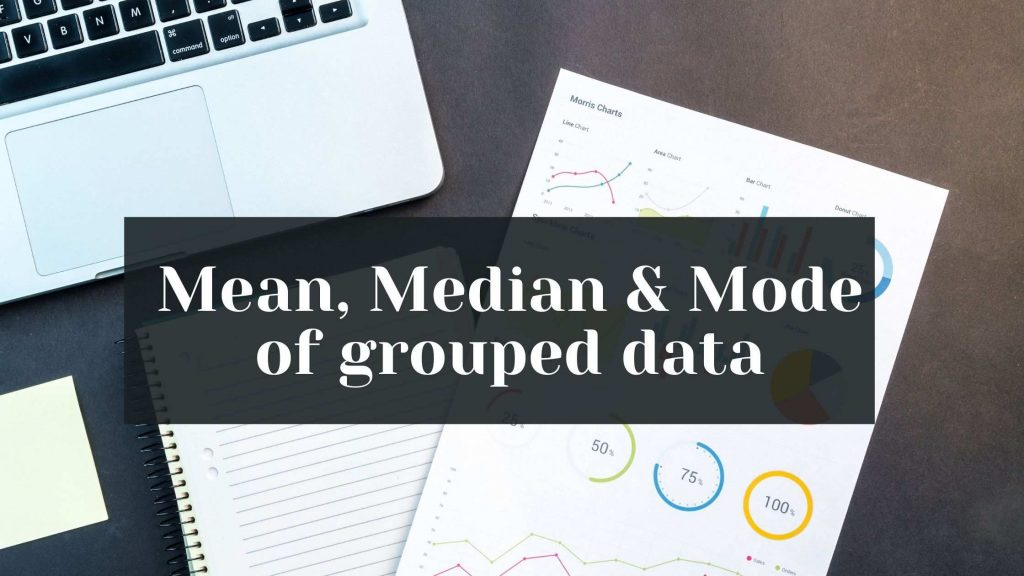Hesap Oluştur

Got stuck on homework? Get your step-by-step solutions from real tutors in minutes! 24/7. Unlimited.

Calculating the mean, median and mode of ungrouped data is a lot easier than calculating them for discrete grouped data. Let’s look at the procedures and techniques involved in calculating them. To learn about mean, median and mode of discrete data, click here.

## Calculating Mean

For a general discrete grouped data, as shown in the figure: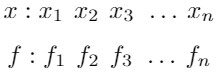The mean is calculated as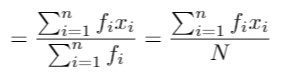where, N is the sum of the frequencies of all elements.

Example 1:
Find the mean for the following data: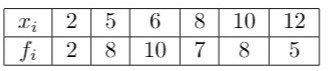Solution: We have been given xis and fis. We know the formula for the mean of discrete grouped data.
The following table illustrates how mean can be calculated in a easy manner,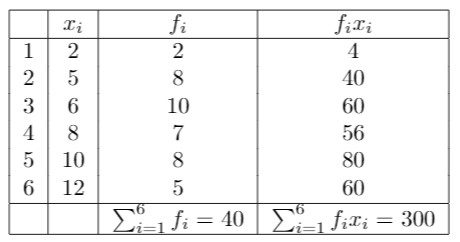Mean =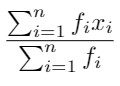= 300/40 = 7.5

## Calculating Median

Calculating median for discrete grouped data is as simple as calculating median for discrete data (ungrouped).
Steps involved in calculating median for discrete data (ungrouped):

• Sort the given data either in ascending order or descending order
• If the total number of elements is odd, then the median is the element in the middle i.e., at 1/2 * (n+1)th position.
• If the total number of elements is even, then the median is the average of the numbers at n/2 and (n/2 + 1)th position numbers.

Median for discrete grouped data is calculated in a very similar way to how we can calculate median for discrete data (ungrouped). Let us go through an example.

Example 2:
Find the median for the following data: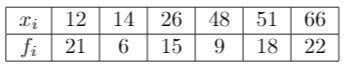Solution:
We can find the median using the cumulative frequency table. The first element with cumulative frequency just greater than or equal to N/2 is the median in case of discrete grouped data.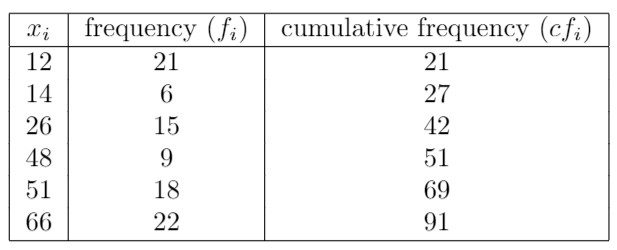Here, we can see that the element 48 is the first element to have cumulative frequency just greater than 45 (N/2 = 91/2). So, the median of this data is 48.

Note: If N is even, and there exists an element whose cumulative frequency is equal to N/2, then median is the average of the element and the next element.
The below example demonstrates this case.
For the discrete grouped data: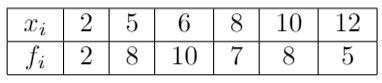The solution table is as follows: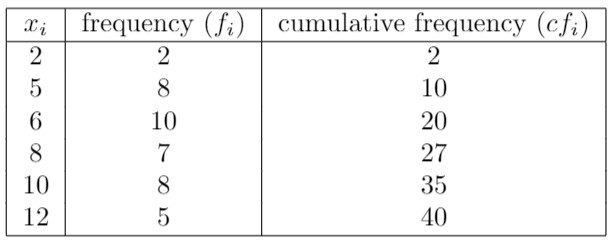Here, we can see that there exists an element (x3=6) with cumulative frequency equal to 20 (40/2). Hence, the median in this case is the average of 6 and 8, which is equals to 7.

## Calculating Mode

Mode calculation for discrete grouped data, is much easier than mean or median calculation. The element with highest frequency is the mode of the data. If two elements have the highest frequency, then the data is considered to be having two modes and is called bimodal data. Similarly, if more than 2 elements have highest frequency, then the data is called multimodal data.

Example 3:
Find the mode of the following data?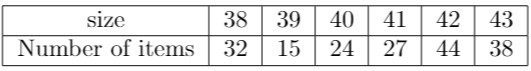Solution:
From the above table, it is observable that the size 42 is the entry with highest frequency, 44.